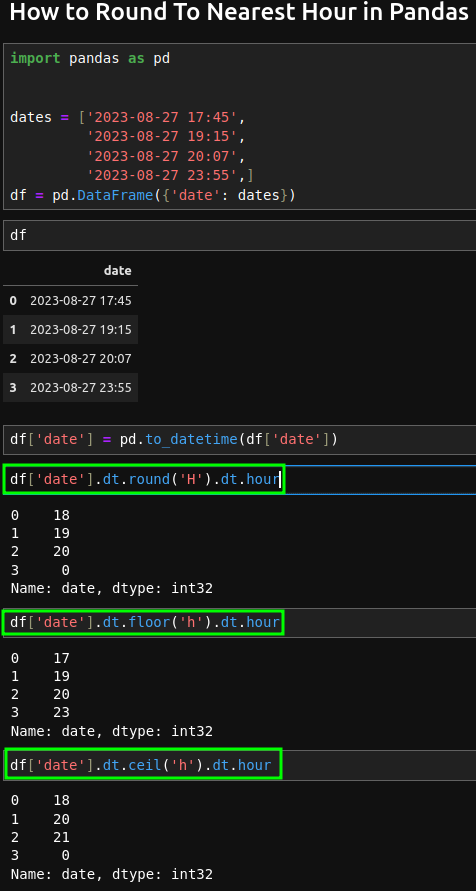To round to closest hour in Pandas datetime column we can several options:

(1) Round to nearest hour

``````df['date'].dt.round('H').dt.hour
``````

(2) floor to closest hour

``````df['date'].dt.floor('h')
``````

(3) ceil to closest hour

``````df['date'].dt.ceil('h')
``````

The image below show the results of 3 options:Let's cover the cases in examples.

## Example

Suppose we have DataFrame with data:

``````import pandas as pd

dates = ['2023-08-27 17:45',
'2023-08-27 19:15',
'2023-08-27 20:07',
'2023-08-27 23:55',]
df = pd.DataFrame({'date': dates})
``````

result:

date
0 2023-08-27 17:45:00
1 2023-08-27 19:15:00
2 2023-08-27 20:07:00
3 2023-08-27 23:55:00

Note: if you need convert string to datetime column by:

``````df['date'] = pd.to_datetime(df['date'])
``````

## Round to closest hour

First we will try to round to closest hour:

``````df['date'].dt.round('H').dt.hour
``````

This will give us the hour as integer:

``````0	18
1	19
2	20
3 	0
Name: date, dtype: int32
``````

## Floor to nearest hour

Instead of rounding we can floor time to hour. Operation floor means:

rounds a number DOWN to the nearest integer, if necessary, and returns the result.

``````df['date'].dt.floor('h').dt.hour
``````

This will give us the hour as integer:

``````0	17
1	19
2	20
3	23
Name: date, dtype: int32
``````

As you can notice two results differ from the first case.

## Ceil to nearest hour

Instead of rounding we can also ceil time to hour. Operation ceil means:

rounds a number UP to the nearest integer, if necessary, and returns the result.

``````df['date'].dt.ceil('h').dt.hour
``````

This will give us the hour as integer:

``````0	18
1	20
2	21
3 	0
Name: date, dtype: int32
``````

Again we have different results.